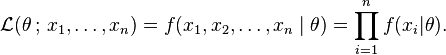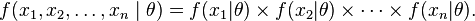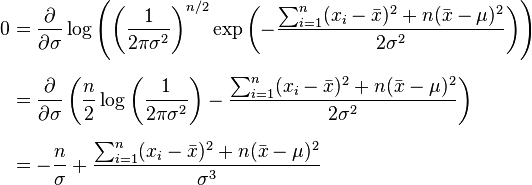Likelihood is a term to replace the following:

The probability of the current observation(s) if the population constant parameter is Theta.

Likelihood=whereF is the pdf for x given theta

Maximum likelihood estimate for the unknown theta:

The constant theta of the population for which the current observation(s) would be most probable to happen.

we find the best model, among all models with different constant thetas, for  which the probability of observations is the most.

We define the maximum, we find for which theta  the derivative of Likelihood f(x|theta) is zero.

\frac{\partial f(x|\theta)) }{\partial \theta}=0

Which will give the same result  as when we solvefor theta.

========================================

Example:

For the normal distribution$\mathcal{N}(\mu, \sigma^2)$ which has probability density function$f(x\mid \mu,\sigma^2) = \frac{1}{\sqrt{2\pi}\ \sigma\ } \exp{\left(-\frac {(x-\mu)^2}{2\sigma^2} \right)},$

the corresponding probability density function for a sample of n independent identically distributed normal random variables (the likelihood) is$f(x_1,\ldots,x_n \mid \mu,\sigma^2) = \prod_{i=1}^{n} f( x_{i}\mid \mu, \sigma^2) = \left( \frac{1}{2\pi\sigma^2} \right)^{n/2} \exp\left( -\frac{ \sum_{i=1}^{n}(x_i-\mu)^2}{2\sigma^2}\right),$

or more conveniently:$f(x_1,\ldots,x_n \mid \mu,\sigma^2) = \left( \frac{1}{2\pi\sigma^2} \right)^{n/2} \exp\left(-\frac{ \sum_{i=1}^{n}(x_i-\bar{x})^2+n(\bar{x}-\mu)^2}{2\sigma^2}\right),$\begin{align} 0 & = \frac{\partial}{\partial \mu} \log \left( \left( \frac{1}{2\pi\sigma^2} \right)^{n/2} \exp\left(-\frac{ \sum_{i=1}^{n}(x_i-\bar{x})^2+n(\bar{x}-\mu)^2}{2\sigma^2}\right) \right) \\[6pt] & = \frac{\partial}{\partial \mu} \left( \log\left( \frac{1}{2\pi\sigma^2} \right)^{n/2} - \frac{ \sum_{i=1}^{n}(x_i-\bar{x})^2+n(\bar{x}-\mu)^2}{2\sigma^2} \right) \\[6pt] & = 0 - \frac{-2n(\bar{x}-\mu)}{2\sigma^2} \end{align}

which will be zero when mean of the population is mean of the sample. Therefore the probability of the observed xs is most when mu is xbar.

fortunately Its expectation value is equal to the parameter μ of the given distribution,$E \left[ \widehat\mu \right] = \mu, \,$

alsoThis is zero whenWhich means the estimator for variance of population sigma considering our observations is variance of the sample.

However,Which means that variance of the sample is biased a little bit.

http://en.wikipedia.org/wiki/Maximum_likelihood

Since 11 April 2023: 224 total views,  2 views today